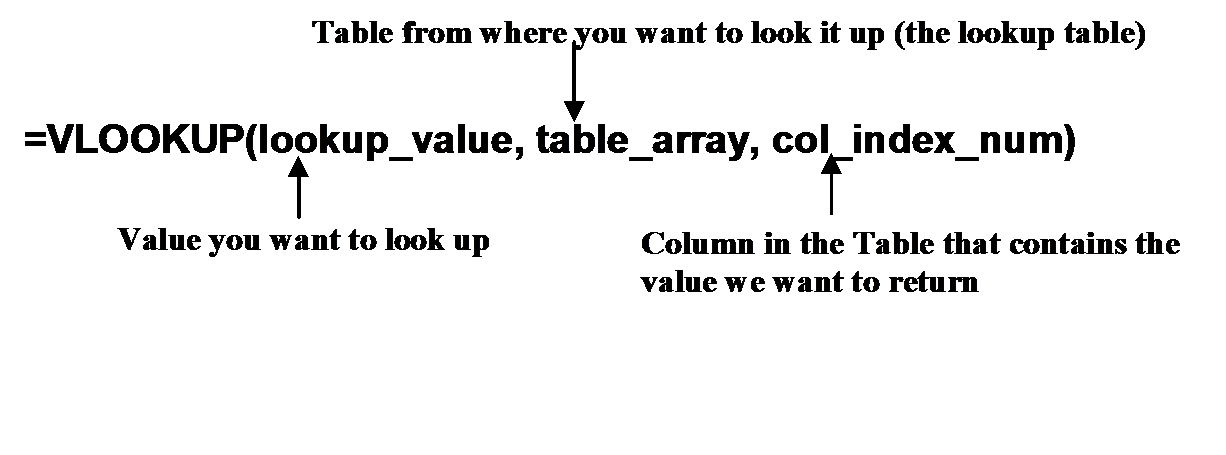# Find Excel data using the VLOOKUP function

Posted by Roger Hyttinen on

The VLOOKUP function allows you to locate data within a defined table, referred to as an array. How it works is that it searches for a value in the leftmost column of a table, and then returns a value in the same row from a column you specify in the table.For instance, suppose in one worksheet we have an order entry list which contains customer name and product ID. In another sheet (our lookup table), we have a list of all products and their corresponding product ID’s. Using the VLOOKUP function, we can insert the name of the product in our order entry list by searching the lookup table for the product name that corresponds to the product ID.

The format of the VLOOKUP function is as follows:In the example above, the first argument, lookup_value would be the cell address of the product_id number – that is to say, the item we want to look up in our table array.
The second argument, table_array is the complete range of our lookup table. This can be a cell address (A1:F131) or a named range (such as mylist). The table must be sorted in alphabetical order by the leftmost column or you will receive an error in your formula. The table array can be located in the same worksheet or in another worksheet.

The third argument, the col_index_num is the column number in the table array to be returned. If the product name column (the column whose value we want to return) was the third column from the left, this argument would be 3.

### Quick Guide: How To Use the VLOOKUP Function

1. Click in the cell where you want to place the formula.
2. Type: =VLOOKUP( to begin the formula.
3. Type the cell address of the value you want to look up.
4. Type a comma ,
5. Type the cell range or the named range of the lookup table (table array)
6. Type a comma ,
7. Type the column number which contains that value that corresponds to lookup_value.
8. Type: ) and then press the Enter Key to confirm the formula.

Note: You can also use the Insert Function button rather than typing in the formula manually.

Looking for more Excel tips? Check out our new book Excel 2016: Up To Speed available as an ebook on Amazon, Kobo, and Apple iBooks

### Share this post

0 comment

Leave a comment

Please note, comments must be approved before they are published.